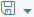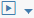# Example Graphs for TensorFlow

Use the TensorFlow-based example graphs in the SAP Data Hub Modeler to build rich machine learning applications.

The SAP Data Hub Modeler provides the following example graphs, which you can use to build productive applications. These graphs are based on the TensorFlow graph described in the tutorial.
• com.sap.ml.tensorflow.trainMnistRepo
• com.sap.ml.tensorflow.evaluateMnistRepo
• com.sap.ml.tensorflow.classifyVideoStream
• com.sap.ml.tensorflow.classifyImages
Executing the com.sap.ml.tensorflow.trainMnistRepo graph
1. Start the SAP Data Hub Modeler.
2. In the navigation pane, select the Graphs tab.
3. In the ML Tensorflow (beta) section, select the Train MNIST Model graph.
4. In the editor toolbar, choose(Save) to save the graph.
5. In the editor toolbar, choose(Run) to execute the graph.

The Status tab in the bottom pane shows the status for the graph execution as running to indicate that the graph is being executed. In the editor, right-click the Terminal operator and choose Open UI. The tool provides information on the accuracy and the model save progress.

Executing the com.sap.ml.tensorflow.evaluateMnistRepo graph

While the training graph is running (or after the graph is stopped), execute the inference graph.

1. In the navigation pane, select the Graphs tab.
2. In the ML Tensorflow (beta) section, select the Infer MNIST Str. Repo graph.
3. In the editor toolbar, choose(Save) to save the graph.
4. In the editor toolbar, choose(Run) to execute the graph.

The Status tab in the bottom pane shows the status for graph execution as running to indicate that the graph is being executed. You can now see inference values (deduced number in the drawn image) of the images and the image paths that are sent to the Terminal operator.

Executing the com.sap.ml.tensorflow.classifyImages graph.
1. In the navigation pane, select the Graphs tab.
2. In the ML Tensorflow (beta) section, select the Classify Images with Inception graph.
3. In the editor toolbar, choose(Save) to save the graph.
4. In the editor toolbar, choose(Run) to execute the graph.

The Status tab in the bottom pane shows the status for graph execution as running to indicate that the graph is being executed.

Executing the com.sap.ml.tensorflow.classifyVideoStream graph.
1. In the navigation pane, select the Graphs tab.
2. In the ML Tensorflow (beta) section, select the Classify Video graph.
3. In the editor toolbar, choose(Save) to save the graph.
4. In the editor toolbar, choose(Run) to execute the graph.

The Status tab in the bottom pane shows the status for graph execution as running to indicate that the graph is being executed.